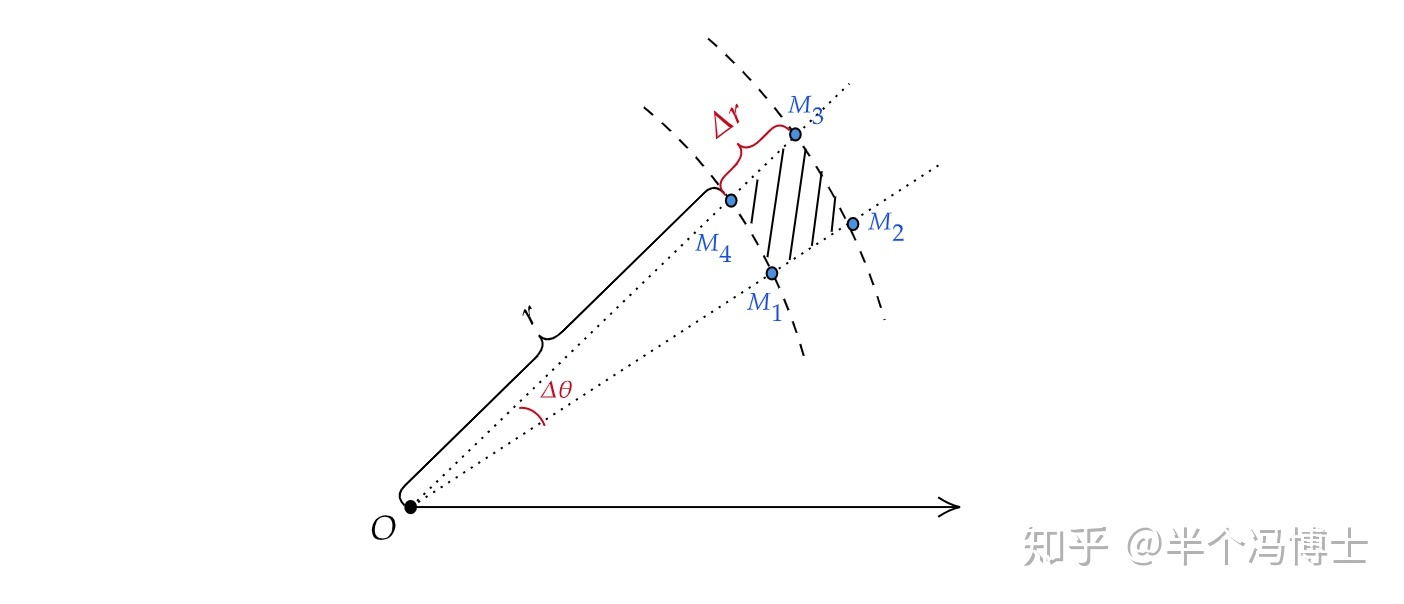# 高斯积分的求解简明笔记

## 【解析】

$\displaystyle I = \int_{-\infty}^{+\infty}e^{-x^2}\text{d}x$

$\displaystyle x^2+y^2=r^2, \text{d}x\text{d}y=r\text{d}r\text{d}\theta$，其中 $\displaystyle r\in\left[0,+\infty\right), \theta\in\left[0,2\pi\right]$

$\displaystyle I^2 = \int_{0}^{2\pi}\int_{0}^{+\infty}e^{-r^2}r\text{d}r\text{d}\theta$

$\displaystyle I^2 = -\frac{1}{2}\times 2\pi\int_{0}^{+\infty}e^{-r^2}\text{d}(-r^2) = -\pi\cdot e^{-r^2}\bigg|_0^{+\infty}=\pi$

## 为什么 dxdy = rdrdθ？\begin{aligned} \text{d}\sigma&=S_{扇M_2OM_3}-S_{扇M_1OM_4}\\&=\frac{1}{2}\left(r+\Delta r\right)^2\cdot\Delta\theta-\frac{1}{2}r^2\cdot\Delta\theta\\&=\frac{1}{2}\left[r^2+2r\Delta r+\left(\Delta r\right)^2-r^2\right]\Delta\theta\\&=\frac{1}{2}\left[2r\Delta r+\left(\Delta r\right)^2\right]\cdot\Delta\theta \end{aligned}

“我这么可爱，请给我钱 o(*^ω^*)o”https://blog.kukmoon.com/ac54c2ee/

Kukmoon谷月

2021年11月11日